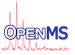OpenMS  2.8.0

`#include <OpenMS/DATASTRUCTURES/Adduct.h>`

[legend]

## Public Member Functions

Default C'tor. More...

C'tor with initial charge. More...

Adduct (Int charge, Int amount, double singleMass, String formula, double log_prob, double rt_shift, const String label="")
C'tor for all members. More...

Adduct operator* (const Int m) const
Increase amount of this adduct by factor. More...

Add two adducts amount if they are equal (defined by equal formula) More...

const IntgetCharge () const

void setCharge (const Int &charge)

const IntgetAmount () const

void setAmount (const Int &amount)

const double & getSingleMass () const

void setSingleMass (const double &singleMass)

const double & getLogProb () const

void setLogProb (const double &log_prob)

const StringgetFormula () const

void setFormula (const String &formula)

const double & getRTShift () const

const StringgetLabel () const

## Private Member Functions

String checkFormula_ (const String &formula)

## Private Attributes

Int charge_
usually +1 More...

Int amount_
number of entities More...

double singleMass_
mass of a single entity More...

double log_prob_
log probability of observing a single entity of this adduct More...

String formula_
chemical formula (parsable by EmpiricalFormula) More...

double rt_shift_
RT shift induced by a single entity of this adduct (this is for adducts attached prior to ESI, e.g. labeling) More...

String label_
Label for this adduct (can be used to indicate heavy labels) More...

## Friends

std::ostream & operator<< (std::ostream &os, const Adduct &a)
Print the contents of an Adduct to a stream. More...

Comparator. More...

## Constructor & Destructor Documentation

Default C'tor.

C'tor with initial charge.

 Adduct ( Int charge, Int amount, double singleMass, String formula, double log_prob, double rt_shift, const String label = `""` )

C'tor for all members.

## ◆ checkFormula_()

 String checkFormula_ ( const String & formula )
private

## ◆ getAmount()

 const Int& getAmount ( ) const

## ◆ getCharge()

 const Int& getCharge ( ) const

## ◆ getFormula()

 const String& getFormula ( ) const

## ◆ getLabel()

 const String& getLabel ( ) const

## ◆ getLogProb()

 const double& getLogProb ( ) const

## ◆ getRTShift()

 const double& getRTShift ( ) const

## ◆ getSingleMass()

 const double& getSingleMass ( ) const

## ◆ operator*()

 Adduct operator* ( const Int m ) const

Increase amount of this adduct by factor.

Parameters
 m

## ◆ operator+()

Add two adducts amount if they are equal (defined by equal formula)

## ◆ operator+=()

 void operator+= ( const Adduct & rhs )

## ◆ setAmount()

 void setAmount ( const Int & amount )

## ◆ setCharge()

 void setCharge ( const Int & charge )

## ◆ setFormula()

 void setFormula ( const String & formula )

## ◆ setLogProb()

 void setLogProb ( const double & log_prob )

## ◆ setSingleMass()

 void setSingleMass ( const double & singleMass )

## ◆ operator<<

 std::ostream& operator<< ( std::ostream & os, const Adduct & a )
friend

Print the contents of an Adduct to a stream.

## ◆ operator==

 bool operator== ( const Adduct & a, const Adduct & b )
friend

Comparator.

## ◆ amount_

 Int amount_
private

number of entities

## ◆ charge_

 Int charge_
private

usually +1

## ◆ formula_

 String formula_
private

chemical formula (parsable by EmpiricalFormula)

## ◆ label_

 String label_
private

Label for this adduct (can be used to indicate heavy labels)

## ◆ log_prob_

 double log_prob_
private

log probability of observing a single entity of this adduct

## ◆ rt_shift_

 double rt_shift_
private

RT shift induced by a single entity of this adduct (this is for adducts attached prior to ESI, e.g. labeling)

## ◆ singleMass_

 double singleMass_
private

mass of a single entity